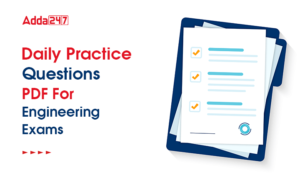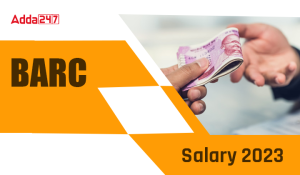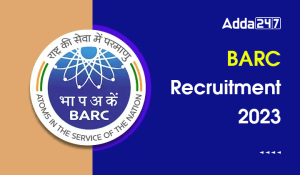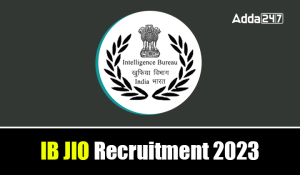Engineering Jobs   »   Mechanical Engineering Quiz

# UPRVUNL’21 ME: Daily Practices Quiz. 21-Aug-2021

UPRVUNL

Quiz: Mechanical Engineering
Exam: UPRVUNL
Topic: Miscellaneous
Each question carries 1 mark
Negative marking: 1/4 mark
Time: 8 Minutes

Q1. Match List-I with List-II and select the correct answer using the codes given below the lists:Codes
A B C D
(a) 4 1 3 2
(b) 1 4 2 3
(c) 4 1 2 3
(d) 1 2 3 4
Q2. A furnace wall is as shown in the figure. The interface temperature Ti will be(a) 560°C
(b) 200°C
(c) 920°C
(d) 1120°C
Q3. Upto the critical radius of insulation
(a) convection heat loss will be less than conduction heat loss
(b) heat flux decrease
(c) added insulation will increase heat loss
(d) added insulation will decrease heat loss

Q4. Generally fins are provided to increase the rate of heat transfer, but fins also act as insulation. Which one of the following non-dimensional numbers decides this factor?
(a) Eckert number
(b) Biot number
(c) Fourier number
(d) Peclet number
L1Difficulty 3
QTags Mechanical
QCereator Paper Maker 10

Q5. Given that
Pr = Prandtl number
Nu = Nusselt Number
Sh = Sherwood number
E = Reynolds number
Sc = Schmidt number
Gr = Grashoff number
(a) Nu = f(Gr, Pr)
(b) Sh = f(Sc, Gr)
(c) Nu = f(Re, Pr)
(d) Sh = f(Re, Sc)
L1Difficulty 3
QTags Mechanical
QCereator Paper Maker 10

Q6. Consider two infinitely long blackbody concentric cylinders with a diameter ratio D_2/D_1 =2. The shape factor for the outer cylinder with itself will be(a) 0
(b) 1/3
(c) 1/2
(d) 1

Solution
S1. Ans. (c)
Sol.
A. Work done in a polytropic process: (p_1 V_1-p_2 V_2)/(n-1)
B. Work done in steady flow process -∫▒Vdp
C. Heat transfer in a reversible adiabatic process: zero
D. Work done is an isentropic process: (p_1 V_1-p_2 V_2)/(γ-1)

S2. Ans. (c)
Sol.
1000/((0.3/3) )=(T_i-120)/((0.3/0.3) )
1000-T_i=0.1 T_i-12
1.1 T_i=1012
T_i=920°C

S3. Ans. (c)
Sol.From the above plot between heat transfer rate versus r, it can be concluded that by providing insulation, up to critical radius heat transfer rate increase and become maximum at critical radius and further insulation decreases the heat transfer rate

S4. Ans. (b)
Sol.
The heat flow rate will be minimum when biot number is equal to unity and will increase when Biot number < 1.

S5. Ans. (b)
Sol.
Sh = f(Sc, Gr)

S6. Ans. (c)
Sol.
A_1 F_12=A_2 F_21
F_21=A_1/A_2 F_12
From geometry
F_12=1
F_21+F_22=11
F_22=1-F_21
F_22=1-A_1/A_2 =1-(πD_1 L)/(πD_2 L)
F_22=1-1/2=1/2

Sharing is caring!

•GATE Result 2023 Out, Download Result, C...
•GATE 2023 Admit Card Out, Download Link ...
•Daily Practice Questions PDF For Enginee...
•BARC Previous Year Question Papers, Down...
•BARC Salary 2023, Check Perks and Allowa...
•BARC Recruitment 2023, Notification, Vac...
•ISRO Scientist Recruitment 2023 Out, App...
•IB JIO Recruitment 2023 Out, Direct Link...